# Quiz 3: Motion in Two Dimensions

Physics & Astronomy

Solution: Neither the x component of the vector nor the y component of the vector can be greater than the magnitude of the vector. Because, we calculate the magnitude of the vector by using the Pythagoras theorem, that is the square root of the sum of the square of each component of the vector gives the magnitude. Let us explain it with an example. Consider a vector A with its components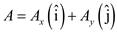The magnitude of this vector is,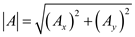From this equation, we can conclude that x component of the vector nor the y component of the vector can be greater than the magnitude of the vector.

The airplane moves with the speed v and makes an angle with the horizontal axis. Then the speed of the airplane resolved into horizontal and vertical components. The resolution of the speed of airplane is as shown in the figure.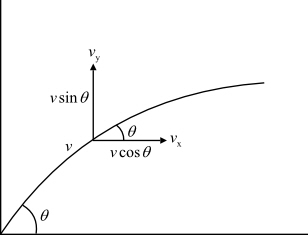In the figure,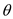is the angle made by the speed of the airplane, v is the actual speed of the airplane,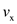is the horizontal component of speed of the airplane, and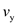is the vertical component of speed of the airplane. (a) The horizontal component of speed of the airplane is,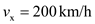From the figure, the horizontal component of speed of the airplane is,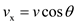Here,is the horizontal component of speed of the airplane, v is the actual speed of the airplane, andis the angle made by the speed of the airplane. Rewrite the equationfor v.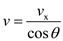Substitute 200 km/h forand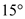forin the above equation solve for v.Therefore, the actual speed of the airplane is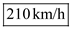. (b) The magnitude of the vertical component of speed of the airplane is,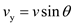Here,is the vertical component of speed of the airplane. Substitute 210 km/h for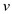andforin the equationand solve for.Therefore, the magnitude of the vertical component of speed of the airplane is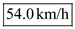.

Consider a velocity vector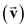in the first quarter of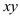plane. If this velocity vector made an anglewith positive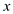axis in counter clockwise direction, the components of velocity alongaxis and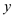axis are shown in the below figure.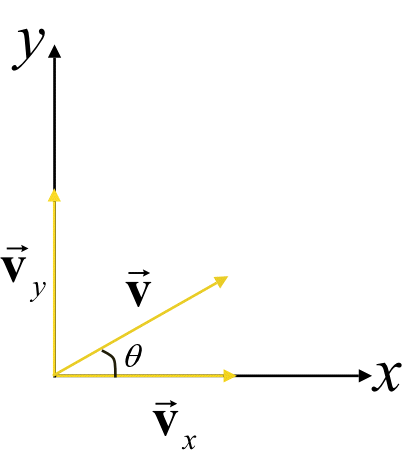From the above figure,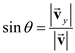Rearrange the above equation for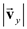as follows: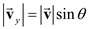Similarly, the cosine of the angle from the above figure is,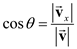Rearrange the above equation for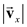as follows: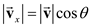From this equation,component of velocity associated with cosine. Therefore, the correct option is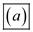.

There is no answer for this question